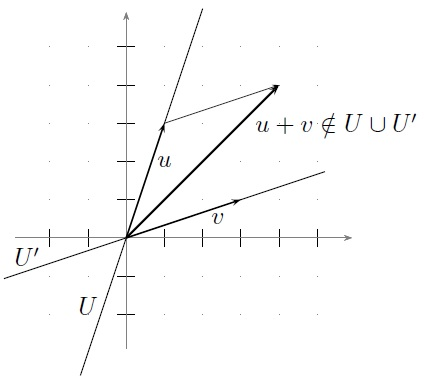$$\newcommand{\id}{\mathrm{id}}$$ $$\newcommand{\Span}{\mathrm{span}}$$ $$\newcommand{\kernel}{\mathrm{null}\,}$$ $$\newcommand{\range}{\mathrm{range}\,}$$ $$\newcommand{\RealPart}{\mathrm{Re}}$$ $$\newcommand{\ImaginaryPart}{\mathrm{Im}}$$ $$\newcommand{\Argument}{\mathrm{Arg}}$$ $$\newcommand{\norm}{\| #1 \|}$$ $$\newcommand{\inner}{\langle #1, #2 \rangle}$$ $$\newcommand{\Span}{\mathrm{span}}$$

# 4.4: Sums and direct sum

$$\newcommand{\vecs}{\overset { \rightharpoonup} {\mathbf{#1}} }$$ $$\newcommand{\vecd}{\overset{-\!-\!\rightharpoonup}{\vphantom{a}\smash {#1}}}$$$$\newcommand{\id}{\mathrm{id}}$$ $$\newcommand{\Span}{\mathrm{span}}$$ $$\newcommand{\kernel}{\mathrm{null}\,}$$ $$\newcommand{\range}{\mathrm{range}\,}$$ $$\newcommand{\RealPart}{\mathrm{Re}}$$ $$\newcommand{\ImaginaryPart}{\mathrm{Im}}$$ $$\newcommand{\Argument}{\mathrm{Arg}}$$ $$\newcommand{\norm}{\| #1 \|}$$ $$\newcommand{\inner}{\langle #1, #2 \rangle}$$ $$\newcommand{\Span}{\mathrm{span}}$$ $$\newcommand{\id}{\mathrm{id}}$$ $$\newcommand{\Span}{\mathrm{span}}$$ $$\newcommand{\kernel}{\mathrm{null}\,}$$ $$\newcommand{\range}{\mathrm{range}\,}$$ $$\newcommand{\RealPart}{\mathrm{Re}}$$ $$\newcommand{\ImaginaryPart}{\mathrm{Im}}$$ $$\newcommand{\Argument}{\mathrm{Arg}}$$ $$\newcommand{\norm}{\| #1 \|}$$ $$\newcommand{\inner}{\langle #1, #2 \rangle}$$ $$\newcommand{\Span}{\mathrm{span}}$$

Throughout this section, $$V$$ is a vector space over $$\mathbb{F}$$, and $$U_1 , U_2 \subset V$$ denote subspaces.

Definition 4.4.1: (subspace) sum

Let $$U_1 , U_2 \subset V$$ be subspaces of $$V$$ . Deﬁne the (subspace) sum of $$U_1$$Figure 4.4.1: The union $$U \cup U^\prime$$ of two subspaces is not necessarily a subspace.

and $$U_2$$ to be the set

$U_1 + U_2 = \{u_1 + u_2 | u_1 \in U_1 , u_2 \in U_2 \}. \tag{4.4.1}$

Check as an exercise that $$U_1 + U_2$$ is a subspace of $$V$$ . In fact, $$U_1 + U_2$$ is the smallest subspace of $$V$$ that contains both $$U_1$$ and $$U_2$$ .

Example 4.4.2. Let

$U_1 = \{(x, 0, 0) \in \mathbb{F}^3 | x \in \mathbb{F}\}, \\ U_2 = \{(0, y, 0) \in \mathbb{F}^3 | y \in \mathbb{F}\}.$

Then

$U_1 + U_2 = \{(x, y, 0) \in \mathbb{F}^3 | x, y \in \mathbb{F}\}. \tag{4.4.2}$

If, alternatively, $$U_2 = \{(y, y, 0) \in \mathbb{F}^3 | y \in \mathbb{F}\}$$, then Equation (4.4.2) still holds.

If $$U = U_1 +U_2$$ , then, for any $$u \in U$$, there exist $$u_1 \in U_1$$ and $$u_2 \in U_2$$ such that $$u = u_1 +u_2.$$

If it so happens that $$u$$ can be uniquely written as $$u_1 + u_2$$ , then $$U$$ is called the direct sum of $$U_1$$ and $$U_2.$$

Definition 4.4.3: Direct Sum

Suppose every $$u \in U$$ can be uniquely written as $$u = u_1 + u_2$$ for $$u_1 \in U_1$$ and $$u_2 \in U_2$$ . Then we use

$U = U_1 \oplus U_2$

to denote the direct sum of $$U_1$$ and $$U_2.$$

Example 4.4.4. Let
$U_1 = \{(x, y, 0) \in \mathbb{R}^3 | x, y \in \mathbb{R}\}, \\ U_2 = \{(0, 0, z) \in \mathbb{R}^3 | z \in \mathbb{R}\}.$
Then $$\mathbb{R}^3 = U_1 \oplus U_2$$. However, if instead
$U_2 = \{(0, w, z) | w, z \in \mathbb{R}\},$
then $$\mathbb{R}^3 = U_1 + U_2$$ but is not the direct sum of $$U_1$$ and $$U_2$$ .
Example 4.4.5. Let
$U_1 = \{p \in \mathbb{F}[z] | p(z) = a_0 + a_2 z^2 + \cdots + a_{2m}z^{2m} \}, \\ U_2 = \{p \in \mathbb{F}[z] | p(z) = a_1z + a_3z^3 + \cdots + a_{2m+1}z^{2m+1} \}.$
Then $$\mathbb{F}[z] = U_1 \oplus U_2.$$

Proposition 4.4.6. Let $$U_1 , U_2 \subset V$$ be subspaces. Then $$V = U_1 \oplus U_2$$ if and only if the following two conditions hold:

1. $$V = U_1 + U_2;$$
2. If $$0 = u_1 + u_2$$ with $$u_1 \in U_1$$ and $$u_2 \in U_2$$ , then $$u_1 = u_2 = 0.$$

Proof.
$$(“\Rightarrow”)$$ Suppose $$V = U_1 \oplus U_2$$. Then Condition 1 holds by deﬁnition. Certainly $$0 = 0 + 0$$, and, since by uniqueness this is the only way to write $$0 \in V$$ , we have $$u_1 = u_2 = 0$$.

$$(“\Leftarrow”)$$ Suppose Conditions 1 and 2 hold. By Condition 1, we have that, for all $$v \in V$$ , there exist $$u_1 \in U_1$$ and $$u_2 \in U_2$$ such that $$v = u_1 + u_2$$ . Suppose $$v = w_1 + w_2$$ with $$w_1 \in U_1$$ and $$w_2 \in U_2$$ . Subtracting the two equations, we obtain

$0 = (u_1 − w_1 ) + (u_2 − w_2 ),$

where $$u_1 − w_1 \in U_1$$ and $$u_2 − w_2 \in U_2$$. By Condition 2, this implies $$u_1 − w_1 = 0$$ and $$u_2 − w_2 = 0$$, or equivalently $$u_1 = w_1$$ and $$u_2 = w_2$$ , as desired.

Proposition 4.4.7. Let $$U_1 , U_2 \subset V$$ be subspaces. Then $$V = U_1 \oplus U_2$$ if and only if the following two conditions hold:

1. $$V = U_1 + U_2;$$
2. $$U_1 \cap U_2 = \{0\}.$$

Proof.
$$(“\Rightarrow”)$$ Suppose $$V = U_1 \oplus U_2$$. Then Condition 1 holds by deﬁnition. If $$u \in U_1 \cap U_2$$ , then $$0 = u + (−u)$$ with $$u \in U_1$$ and $$−u \in U_2$$ (why?). By Proposition 4.4.6, we have $$u = 0$$ and $$−u = 0$$ so that $$U_1 \cap U_2 = \{0\}.$$

$$(“\Leftarrow”)$$ Suppose Conditions 1 and 2 hold. To prove that $$V = U_1 \oplus U_2$$ holds, suppose that

$0 = u_1 + u_2, \rm{~where~} u_1 \in U_1 \rm{~and~} u_2 \in U_2. \tag{4.3}$

By Proposition 4.4.6, it suﬃces to show that $$u_1 = u_2 = 0$$. Equation (4.3) implies that $$u_1 = −u_2 \in U_2$$. Hence $$u_1 \in U_1 \cap U_2$$, which in turn implies that $$u_1 = 0$$. It then follows that $$u_2 = 0$$ as well.

Everything in this section can be generalized to m subspaces $$U_1 , U_2 , \ldots U_m,$$ with the notable exception of Proposition 4.4.7. To see, this consider the following example.

Example 4.4.8. Let

$U_1 = \{(x, y, 0) \in \mathbb{F}^3 | x, y \in \mathbb{F}\}, \\ U_2 = \{(0, 0, z) \in \mathbb{F}^3 | z \in \mathbb{F}\}, \\ U_3 = \{(0, y, y) \in \mathbb{F}^3 | y \in \mathbb{F}\}.$

Then certainly $$\mathbb{F}^3 = U_1 + U_2 + U_3$$ , but $$\mathbb{F}^3 \neq U_1 \oplus U_2 \oplus U_3$$ since, for example,

$(0, 0, 0) = (0, 1, 0) + (0, 0, 1) + (0, −1, −1).$

But $$U_1 \cap U_2 = U_1 \cap U_3 = U_2 \cap U_3 = \{0\}$$ so that the analog of Proposition 4.4.7 does not hold.

## Contributors

Both hardbound and softbound versions of this textbook are available online at WorldScientific.com.

4.4: Sums and direct sum is shared under a not declared license and was authored, remixed, and/or curated by Isaiah Lankham, Bruno Nachtergaele, & Anne Schilling.